## How to find nth occurrence (position) of a character in text string in Excel?

For example, there is a long sentence in Cell A1, see the following screenshot. And now you need to find the 3rd occurrence or position of Character "c" from the text string in Cell A1. Of course, you can count the characters one by one, and get the exact position result. However, here we are going to introduce some easy tips to find the nth occurrence or position of specific character from text string in a cell.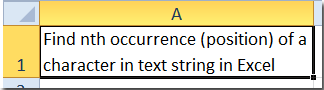#### Find nth occurrence (position) of a character in a Cell with Find formula

There are two Find formulas can help you find the nth occurrence or position of specific character from text string in a cells quickly.

The following formula will show you how to find the 3rd occurrence of "c" in Cell A1.

Find Formula 1

In a blank cell, enter the formula =FIND("c",A1,FIND("c",A1)+2).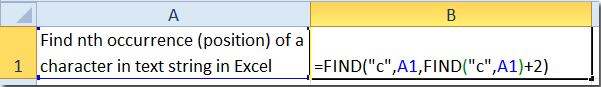And then press the Enter key. The position of the third letter “c” has been displayed.

Note: You can change the 2 in the formula based on your needs. For example, if you want to find the fourth position of "c", you can change the 2 to 3. And if you want to find the first position of "c", you shuold change 2 to 0.

Find formula 2

In a blank cell, enter the formula =FIND(CHAR(1),SUBSTITUTE(A1,"c",CHAR(1),3)), and press Enter key.

Note: The "3" in the formula means the third "c", you can change it based on your needs.

#### Count times a word appears in an excel cell

If a word appears several times in a cell which needed to be count, usually, you may count them one by one. But if the word appears hundreds of times, the manually counting is troublesome. The Count times a word appears function in Kutools for Excel's Formula Helper group can quickly calculate the count of times a word appear in a cell. Free trial with full features in 30 days!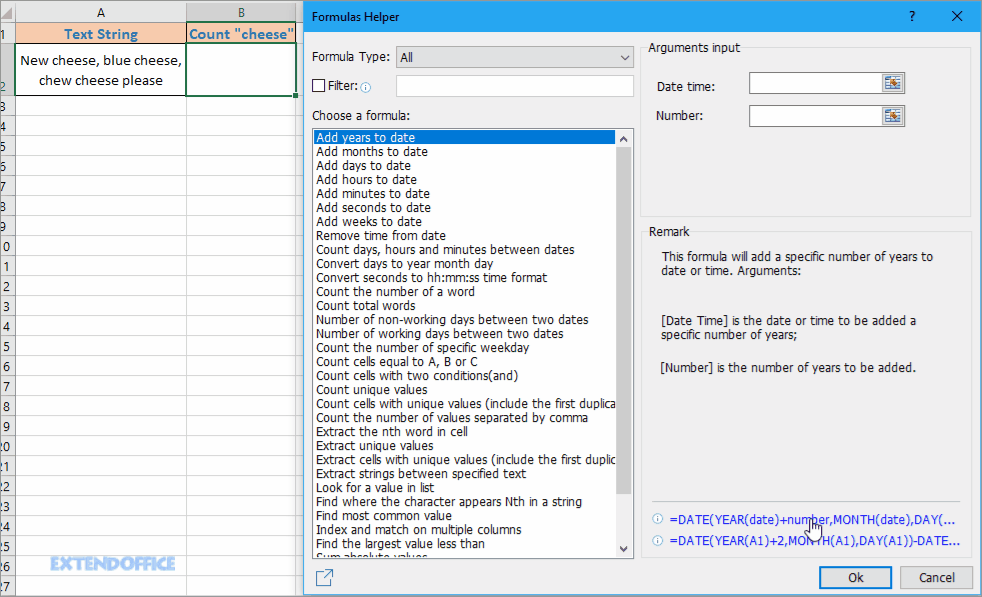Kutools for Excel: with more than 300 handy Excel add-ins, free to try with no limitation in 30 days.

#### > Find nth occurrence (position) of a character in a Cell with VBA

Actually, you can apply the VB macro to find nth occurrence or position of a specific character in one cell easily.

Step 1: Hold down the ALT + F11 keys, and it opens the Microsoft Visual Basic for Applications window.

Step 2: Click Insert > Module, and paste the following macro in the Module Window.

VBA: Find the nth position of a charater.

``````Function FindN(sFindWhat As String, _
sInputString As String, N As Integer) As Integer
Dim J As Integer
Application.Volatile
FindN = 0
For J = 1 To N
FindN = InStr(FindN + 1, sInputString, sFindWhat)
If FindN = 0 Then Exit For
Next
End Function``````

Step 3: Now if you want find the exact occurrence of position of third "c" in Cell A1, please enter the formula of =FindN("c",A1,3), and press the Enter key. Then it will return the exact position in the specific cell at once.

#### Find nth occurrence (position) of a character in a Cell with Kutools for Excel

If you like neither of formula and VBA, you can try a handy tool – Kutools for Excel, with its Formula groups, you can find a utility – Find nth occurrence of a character to quickly return the nth position of a character in a cell.

 with more than 300 handy functions, makes your jobs more easier. Free Downloadfree full-featured in 30 days

After Kutools for Excel, please do as below:

1. Select a cell you want to return the result and click Kutools > Formula Helper > Formula Helper . See screenshot: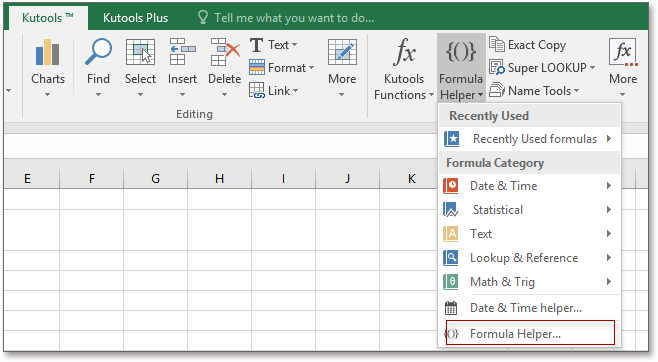2. Then in the popping Formula Helper dialog, do as below:

1) Select Lookup from the drop-down list of Formula Type section;

2) Choose Find where the character appear Nth in a string in Choose a formula section;

3) Select the cell which contains the string you use, then type the specified character and nth occurrence in to the textboxes in the Arguments input section.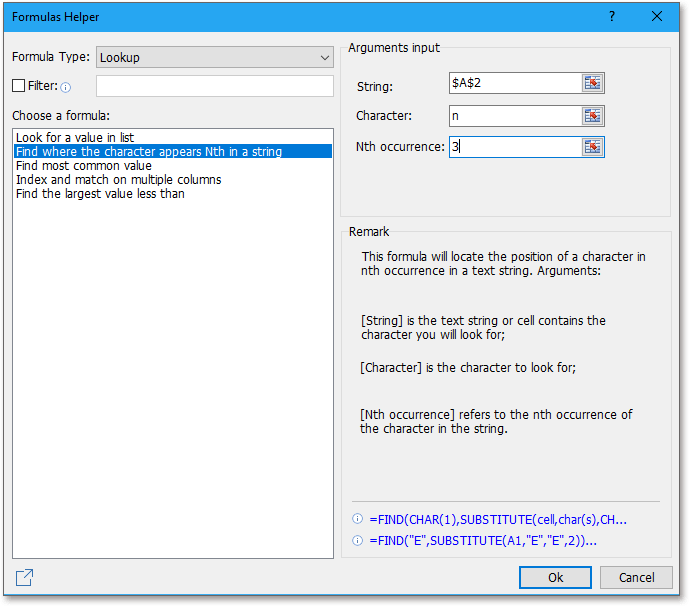3. Click Ok. And you get the position of nth occurrence of a character in a string.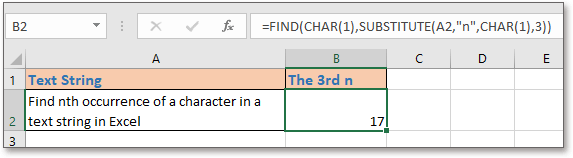### Best Office Productivity Tools

Supercharge Your Spreadsheets： Experience Efficiency Like Never Before with Kutools for Excel

 Popular Features: Find/Highlight/Identify Duplicates   |  Delete Blank Rows   |  Combine Columns or Cells without Losing Data   |   Round without Formula ... Super Lookup: Multiple Criteria VLookup  |   Multiple Value VLookup  |   VLookup Across Multiple Sheets   |   Fuzzy Lookup .... Advanced Drop-down List: Quickly Create Drop Down List   |  Dependent Drop Down List   |  Multi-select Drop Down List .... Column Manager: Add a Specific Number of Columns   |   Move Columns   |   Unhide Columns   |   Compare Columns to Select Same & Different Cells ... Featured Features: Grid Focus   |  Design View   |   Big Formula Bar   |  Workbook & Sheet Manager   |  Resource Library (Auto Text)   |  Date Picker   |  Combine Worksheets   |  Encrypt/Decrypt Cells   |  Send Emails by List   |  Super Filter   |   Special Filter (filter bold/italic/strikethrough...) ... Top 15 Toolset:  12 Text Tools (Add Text, Remove Characters, ...)   |   50+ Chart Types (Gantt Chart, ...)   |   40+ Practical Formulas (Calculate age based on birthday, ...)   |   19 Insertion Tools (Insert QR Code, Insert Picture from Path, ...)   |   12 Conversion Tools (Numbers to Words, Currency Conversion, ...)   |   7 Merge & Split Tools (Advanced Combine Rows, Split Cells, ...)   |   Many More...

Kutools for Excel boasts over 300 features, ensuring that what you need is just a click away...

Supports Office/Excel 2007-2021 & newer, including 365   |   Available in 44 languages   |   Enjoy a full-featured 30-day free trial.#### Office Tab Brings Tabbed interface to Office, and Make Your Work Much Easier

• Enable tabbed editing and reading in Word, Excel, PowerPoint, Publisher, Access, Visio and Project.
• Open and create multiple documents in new tabs of the same window, rather than in new windows.
• Increases your productivity by 50%, and reduces hundreds of mouse clicks for you every day!No ratings yet. Be the first to rate!
This comment was minimized by the moderator on the site
Your formula to find the Nth occurrence of a character in a string does not work. Using your example, adding 2 to the results of the imbedded find ensures a starting position for the initial find of a position beyond the 1st two c's but changing the +2 to +3 does not yield the position of the 4th "c", it simply starts the search one character deeper into the string and the result is still the 3rd "c". To find the 4th "c" the search must begin beyond the position of the 3rd "c". I look forward to your new solution.
This comment was minimized by the moderator on the site
Hello, David

Yes, as you said, the first formula does not work correctly, you can apply the second formula:
``=FIND(CHAR(1),SUBSTITUTE(A2,"c",CHAR(1),2))``

Note: The number "2" in the formula means the second "c", if you want to get the third, fourth "c", just change the number 2 to 3,4 as you need.
This comment was minimized by the moderator on the site
Find formula1 is imperfect. It will be a great help if it is removed. I have lost lot of time trying for 4th occurance of "a" in "vedanarayanan" Thanks.
This comment was minimized by the moderator on the site
To handle the exception of the formula, you can use same with if and iserro
=IF(ISERR(FIND("c",A1,FIND("c",A1)+2)),FIND("c",A1,FIND("c",A1)+0),FIND("c",A1,FIND("c",A1)+2))
This comment was minimized by the moderator on the site
"Substitute" lets you find the Nth occurrence of a term, so combine it with "find" as follows (where 5=5th occurrence):

=FIND(CHAR(1),SUBSTITUTE(A1,"c",CHAR(1),5))
This comment was minimized by the moderator on the site
Excellent! I am glad I scrolled up to review other comments after posting my own.
This comment was minimized by the moderator on the site
This is the correct answer! please replace this whole article with this one line of code.....
This comment was minimized by the moderator on the site
The first formula is completely useless and wrong but it works just correct for this example as the first and second "c"s are adjacent. The correct formula is:

For 2nd occurence -
=FIND("c",A1,FIND("c",A1)+1)

For 3rd occurence -
=FIND("c",A1,FIND("c",A1,FIND("c",A1)+1)+1) and so on...
This comment was minimized by the moderator on the site
Absolutely agree. The +2, or +3 etc.....entirely depends on the spacing between the n and (n+1) occurences of "c". Thus you would have to manually adjust for every cell if you were trying to copy this formula down a column for instance. Essentially the same as manually counting.

Ex. Let's try to find the 4th occurrence of "c" in cell A1 using the provided formula. Per the article we should use +3 in our nested FIND function to find the 4th occurrence.

Text in Cell A1:

"cab car abc cole cube"

Manually counting, the 4th occurrence is the "c" in cole in the character position of 13.

=FIND("c",A1,FIND("c",A1)+3)

The nested function, FIND("c",A1) will find the first occurrence of "c" in position 1.

Therefore,

FIND("c",A1)+3 = 1+3 = 4

Plugging that back into our formula,

=FIND("c",A1,FIND("c",A1)+3) = FIND("c",A1,1+3) = FIND("c",A1,4)

This new formula will look for the first occurrence of "c" beginning from character position 4. The 4th character in the string is the space just after cab.

Therefore, the formula will find the "c" in car and return the value of 5 for our formula. As we know from manually counting we are looking for 13 as the output.
This comment was minimized by the moderator on the site
The firs formula works correct in my version, in the note, it has explained how to find the third or forth or nth "c".

Note: You can change the 2 in the formula based on your needs. For example, if you want to find the fourth position of "c", you can change the 2 to 3. And if you want to find the first position of "c", you shuold change 2 to 0.
This comment was minimized by the moderator on the site
Try to find out the position of the second c with the first sentence of my above comment as the string using the first formula. You'll get to know how correct it is!
This comment was minimized by the moderator on the site
Hello all,

I have a text in an Excel cell " BY TRANSFER-NEFT*HDFC0000001*N08745987123546*J0032331*KUMAR--"

I need a function to extract just "N08745987123546"

Thanks
This comment was minimized by the moderator on the site
Hello, do you want to extract the numbers after No and the number of numbers are fixed? If so, you can use Kutools for Exccel's Extract text function， type No???？to extract.
This comment was minimized by the moderator on the site
HI ALL,
I have a text in an Excel cell " BY TRANSFER-NEFT*HDHC0065431*N053112345624801*K0038331*krishna--"
I need a function to extract just "N053112345624801"
This comment was minimized by the moderator on the site
Formula 1 doesn't work on fourth, fifth, sixth etc. so (+3,4,5) will always show third position of letter "c" which is 19 second formula is working though.
This comment was minimized by the moderator on the site
Hello all, I have a text in an Excel cell "23 floyd lane, longville,KN 14564" I need a function to extract just "longville" Thanks
This comment was minimized by the moderator on the site
If you must use a function, try: =TRIM(MID(A1,SEARCH(",",A1)+1,SEARCH("!@#",SUBSTITUTE(A1,",","!@#",2))-SEARCH(",",A1)-1)) Otherwise, you might consider Data > Text to Columns > Delimited (using a comma as the separator).
This comment was minimized by the moderator on the site
Try this: =INDEX(SMALL(IF(MID(A1,MATCH(ROW(INDIRECT(1&":"&LEN(A1))),ROW(INDIRECT(1&":"&LEN(A1))),0),1)="c",MATCH(ROW(INDIRECT(1&":"&LEN(A1))),ROW(INDIRECT(1&":"&LEN(A1))),0),""),MATCH(ROW(INDIRECT(1&":"&LEN(A1)-LEN(SUBSTITUTE(A1,"c","")))),ROW(INDIRECT(1&":"&LEN(A1)-LEN(SUBSTITUTE(A1,"c","")))),0)),2) where "c" is the character required and 2 is the position. Note this is an array formula.
This comment was minimized by the moderator on the site
this is simpler: =INDEX(SMALL(IF(MID(A1,ROW(INDIRECT(1&":"&LEN(A1))),1)="c",ROW(INDIRECT(1&":"&LEN(A1))),""),ROW(INDIRECT(1&":"&LEN(A1)-LEN(SUBSTITUTE(A1,"c",""))))),2)
This comment was minimized by the moderator on the site
After some playing around I got the following to find the third instance of X and return the position number. =FIND("X",A1,(FIND("X",A1,FIND("X",A1,1)+1))+1) The VBA module gets my vote though. Much easier to remember
This comment was minimized by the moderator on the site
Sure, but you could nest formulae in the "instance number" parameter indefinitely. Formula 2 obviates VBA, making it the best all-round solution.
This comment was minimized by the moderator on the site
Dear All, I want to select a particulate string form a cell Example:- My Name is Mohit i want to return any value Like mohit, is, Name, My using a function. is there any Function or not
This comment was minimized by the moderator on the site
I think there is no specific function for that, since Excel is not built to handle lists of values in one cell. You can build the function yourself by combining the functions mentioned above with LEFT() and RIGHT(). Or you could use VBscripting of course.
This comment was minimized by the moderator on the site
Find Formula 1 won't work. The third parameter of the Find function is the index where the search should begin (as in: the first x characters of the string are ignored). i.e. if a string contains the letter c at indices 1, 5 and 10 the results are as follows: =FIND("c",A1,1) will find the first c =FIND("c",A1,2) through =FIND("c",A1,5) will find the second c =FIND("c",A1,6) through =FIND("c",A1,10) will find the third c. anything above won't find anything.
This comment was minimized by the moderator on the site
Thank you for finding out this mistake, i'm sorry the formula1 is wrong, the correct formula should be =FIND("c",A1,FIND("c",A1)+2) ,and if you want to find the first letter "c", you just need to change the 2 to 0.
This comment was minimized by the moderator on the site
I am able to find second instance but what about 3rd, 4th and 'n'th. I am not able to get 3rd instance (as well as 4th). I have data which contains 50 to 60 instances. so what should I do?
This comment was minimized by the moderator on the site
sanjeev, as I said two years ago, use Formula 2: =FIND(CHAR(1),SUBSTITUTE("LOOK_IN","LOOK_FOR",CHAR(1),INST_NUM)) Where LOOK_IN is the string (or cell) you're searching in; LOOK_FOR is the string you're searching for; and INST_NUM is the integer instance of LOOK_FOR that you want to identify. Hence, =FIND(CHAR(1),SUBSTITUTE("HELLO WORLD","L",CHAR(1),3) will return 10 (the position of the third "L").
This comment was minimized by the moderator on the site
As DdW indicates, the '+2' or '+0' or what-have-you is a 'hard-coded' character position; you'd have to already know where the "c" was, in order to find it. Formula 2 is spot-on, though: SUBSTITUTE the nth instance of your target string for a unique character that won't otherwise appear in the rest of the text, then FIND that unique character.
There are no comments posted here yet